# Cyclic Code

Cyclic Code is known to be a subclass of linear block codes where cyclic shift in the bits of the codeword results in another codeword. It is quite important as it offers easy implementation and thus finds applications in various systems.

Cyclic codes are widely used in satellite communication as the information sent digitally is encoded and decoded using cyclic coding. These are error-correcting codes where the actual information is sent over the channel by combining with the parity bits.

## Content: Cyclic Code

### Introduction

Cyclic codes are known to be a crucial subcategory of linear coding technique because these offers efficient encoding and decoding schemes using a shift register. These are used in error correction as they can check for double or burst errors. Various other important codes like, Reed Solomon, Golay, Hamming, BCH, etc. can be represented using cyclic codes.

Basically, a shift register and a modulo-2 adder are the two crucial elements considered as building blocks of cyclic encoding. Using a shift register, encoding can be efficiently performed. The fundamental elements of shift registers are flip flops (that acts as a storage unit) and input-output. While the other i.e., a binary adder has two inputs and one output.

### Properties of Cyclic Code

We have mentioned at the beginning itself that cyclic codes fall under the category of linear block codes. Let us now understand on following which properties a code is said to be of cyclic nature.

Property 1: Property of Linearity

According to this property, a linear combination of two codewords must be another codeword.

Suppose we have two codewords Ci and Cj. So, on addingwhere this Cp must also be a codeword.

For example, suppose we have given 3 codewords (110, 101, 011).

So, according to linearity property, the addition of any of the two given codewords must produce the third codeword. Let’s check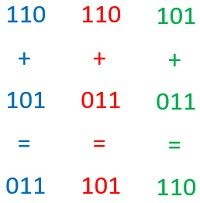Hence, the given code is linear.

Property 2: Property of Cyclic Shifting

According to this property, after a right or left shift in the bits of codewords the resultant code generated must be another codeword.

Suppose, C is a codeword given as:Then after cyclic shiftsHere we have performed the right cyclic shift that has produced these codewords.

For example, consider again those 3 codewords (110, 101, 011) which we considered for linearity property.

So, according to cyclic shifting property, an either right or left shift in the bits of a codeword must generate another codeword.

110: shifting the bits towards the right will provide 011.

101: a right shift in the bits of this codeword will give 110.

011: right shifting of these bits will provide 101.

Hence, it is clear that shifting the bits of the codewords gives rise to another codeword thus cyclic shifting property is verified.

Codes that follow both linearity, as well as cyclic shifting, are called cyclic codes.

It is to be noted here that if a codeword has all 0’s then it is called a valid codeword. But at the same time, 0 is regarded as a necessary condition and not a sufficient condition.

## Example of Cyclic Code

We have discussed in linear block codes as well that a linear codeword is generally given as c(n,k). Here n represents the total bits in the codeword and k denotes the message bits. Thus, the parity bits are (n-k). Suppose we are given a code (7,4) then on comparing with general format the codeword will have 7 bits and the actual message bits are 4 while rest 3 are parity bits.

A cyclic codeword is given as:Then the codeword polynomial will be represented as: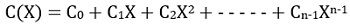For a given codeword C = , the codeword polynomial will be given as:

C(X) = 1*X0 + 0*X1 + 1*X2 + 1*X3

C(X) = X3 + X2 + 1

In a similar way, for any message codeword m, the message polynomialAnd generator polynomialCodewords are classified as systematic and non-systematic codewords.

A systematic codeword is one in which the parity bits and message bits are present in separated forms.

C = [parity bit, message bits]

But a non-systematic codeword is the one in which the message and parity bits exist in intermixed format and cannot be separated just by noticing the initial and final bits.

Let us now understand the coding and decoding of codewords using cyclic code.

### Encoding

Non-Systematic Cyclic Encoding: Consider the message signal given as:

m = 

Thus,

M(X) = 1*X0 + 1*X1 + 1*X2 + 0*X3

M(X) = X2 + X + 1

with generator polynomial G(X) = X3 + X + 1

For non-systematic code, the codeword is given as:C(X) = (X2 + X + 1) (X3 + X + 1)

C(X) = X5 + X3 + X2 + X4 + X2 + X + X3 + X + 1

Here modulo 2 addition will be performed and in modulo 2 addition, the sum of 2 similar bits results in 0.

C(X) = X5 + X3 + X2 + X4 + X2 + X + X3 + X + 1

Thus, X3, X2 and X will get cancelled. So,

C(X) = 1 + X4 + X5

Hence, from the above codeword polynomial, the codeword will be:

C = 

From the codeword bits, we can clearly interpret that the encoded codeword contains the message and parity bits in an intermixed pattern. Thus, is a non-systematic codeword and direct determination of message bits is not possible.

Systematic Cyclic Encoding: Consider another message signal:

m = 

So, message polynomial will be:

M(X) = 1 + X2 + X3

While the generator polynomial G(X) = X3 + X + 1

The equation for determining 7 bits codeword for systematic code is given as:: P(X) represents the parity polynomial and is given by:So, to construct the systematic codeword first we have to determined P(X).

Since n= 7 and k = 4Therefore,Hence, the obtained value of P(X) = 1

Now, substituting the values in codeword polynomial equation,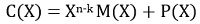C(X) = X3 (X3 + X2 + 1) + 1

C(X) = 1 + X3 + X5 + X6

Hence, the codeword for the above code polynomial will be:

C = 

So, here the first 3 bits are parity bits and the last four bits are message bits. And we can cross-check that we have considered  as the message bits and parity polynomial remainder was 1 i.e., code .

Hence, in this way encoding of non-systematic and systematic codewords is performed.

### Decoding

To understand how the detection of cyclic code takes place. Consider R(X) as received polynomial, C(X) as the encoded polynomial, G(X) to be generator polynomial, and E(X) as error polynomial.

Syndrome or error involved during transmission is given as:Since R(X) is a combination of encoded polynomial and error polynomial thus above equation can be written as:Here, if the obtained remainder is 0 then there will be no error and if the obtained remainder is not 0 then there will be an error that needs to be corrected.This is so because actually coded bit does not contain error thus syndrome is not required to be calculated.

Now, let us check for error polynomial. So, consider the error table given below where we have assumed a single error in each of the bits of the code:So, now using the syndrome for error polynomial formula: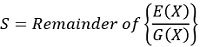We will determine, the erroneous bit. Consider the generator polynomial G(X) = X3 + X + 1 and dividing each error polynomial with it.Now, tabulating it,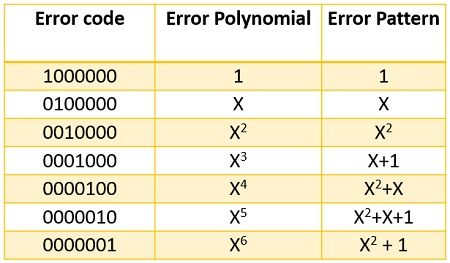Consider an example where transmitted codeword is  received code, r(n,k) = . Hence,

R(X) = 1 + X2 + X5

Now, let us check for the syndrome, by dividing the received polynomial R(X) by the generator polynomial G(X).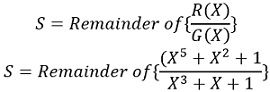We will get,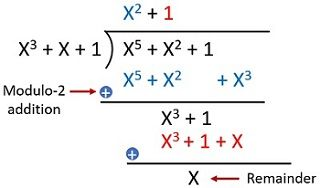S = X ≠ 0

This means the received codeword contains an error. From the tabular representation, it is clear that X has an error code . Thus, this represents an error in the second bit of the received code.

Hence, by using the same approach we can perform encoding and decoding using cyclic code.